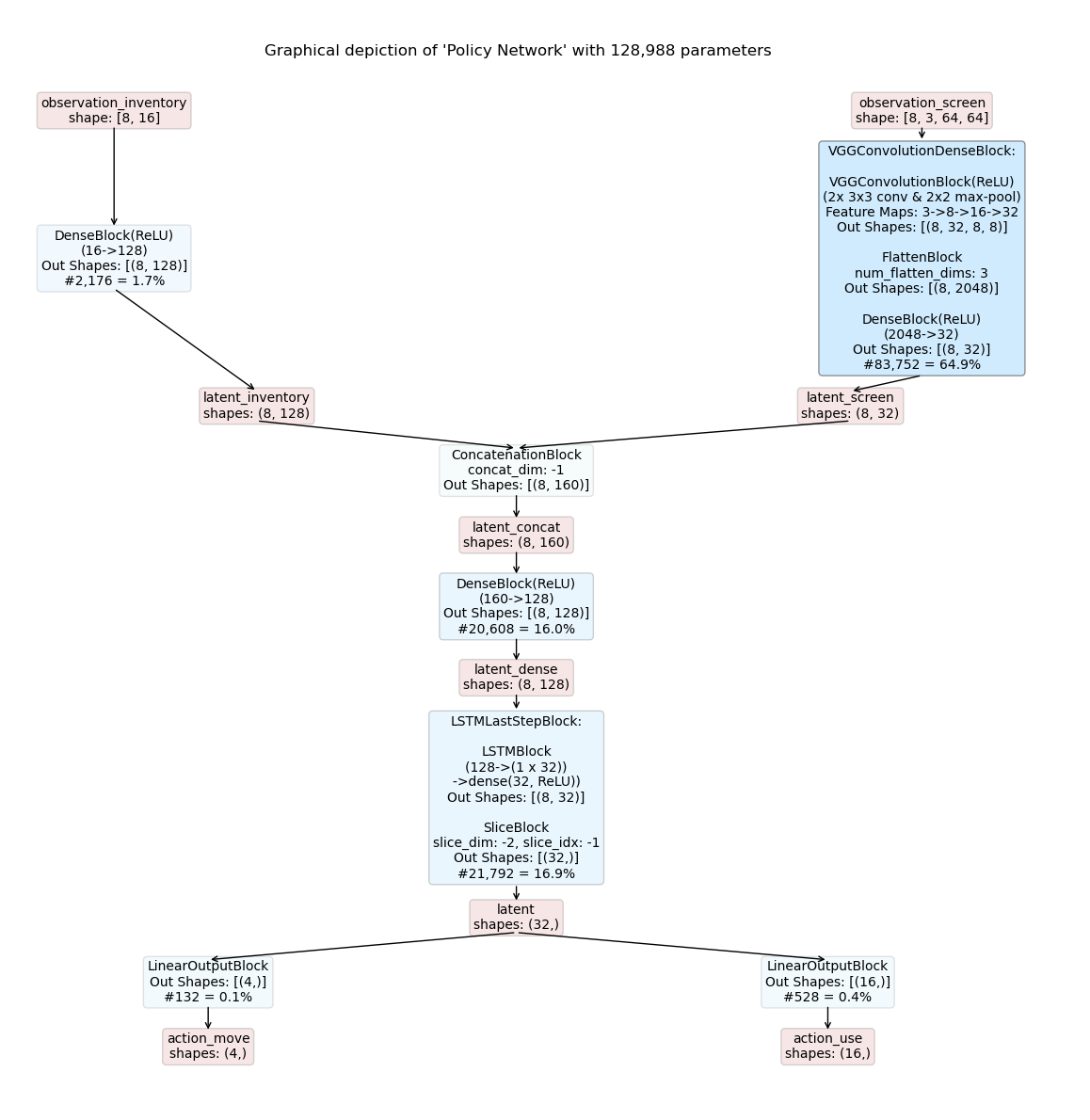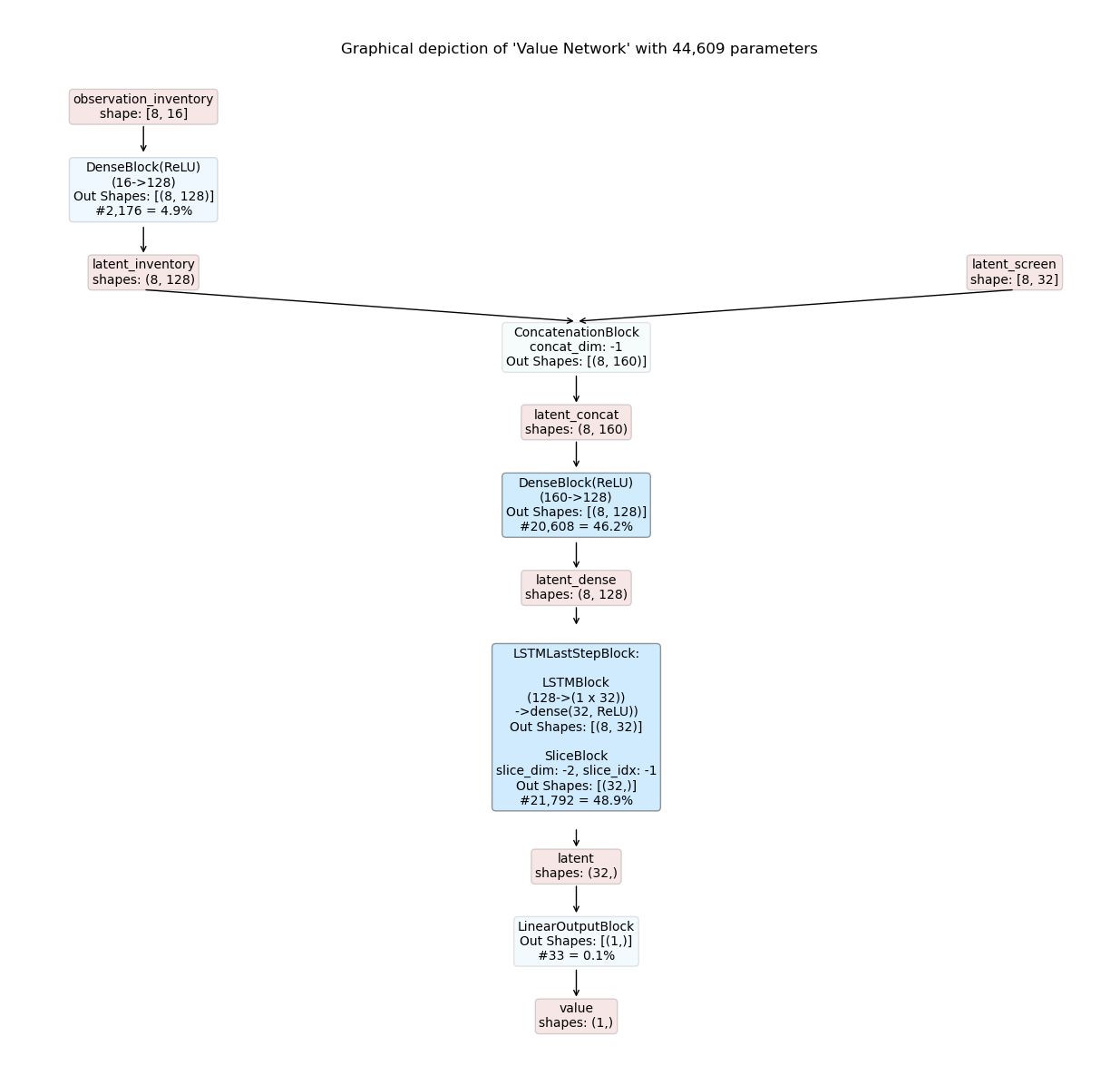Working with Custom Models¶

The Maze custom model composer enables us to explicitly specify application specific models directly in Python. Models can be either written with Maze perception blocks or with plain PyTorch as long as they inherit from Pytorch’s nn.Model.

As such models can be easily created, and even existing models from previous work or well known papers can be easily reused with minor adjustments. However, we recommend to create models using the predefined perception blocks in order to speed up writing as well as to take full advantage of features such as shape inference and graphical rendering of the models.

On this page we cover the features and general working principles. Afterwards we demonstrate the custom model composer with three examples:

List of Features¶

The custom model composer supports the following features:

• Specify complex models directly in Python.

• Supports shape inference and shape checks for a given observation space when relying on Maze perception blocks.

• Reuse existing PyTorch nn.Models with minor modifications.

• Stores a graphical rendering of the networks if the InferenceBlock is utilized.

• Custom weight initialization and action head biasing.

• Custom shared embedding between actor and critic.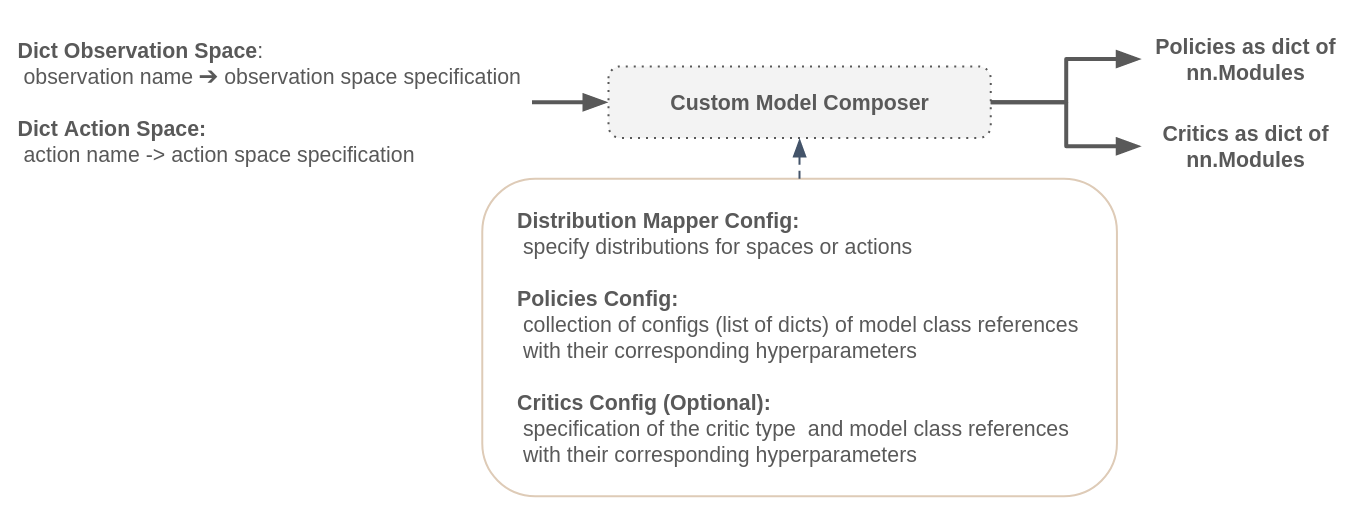The Custom Models Signature¶

The constraints we impose on any model used in conjunction with the custom model composer are threefold: fist, the network class has to adhere to PyTorch’s nn.Model and implement the forward method. Second, a custom network class requires specified constructor arguments depending on the type of network (policy, state critic, …). And lastly the model has to return a dictionary when calling the forward method.

Policy Networks require the constructor arguments obs_shapes and action_logits_shapes. When models are built in the the custom model composer these two arguments are passed to the model constructor in addition to any other arbitrary arguments specified. obs_shapes is a dictionary, mapping observation names to their corresponding shapes. Similarly action_logits_shapes is a dictionary that maps action names to their corresponding action logits shapes. Both, observation and action logits shapes are automatically inferred in the model composer.

• implement nn.Model

• constructor arguments: obs_shapes and action_logits_shapes

• return type of forward method: Here the forward method has to return a dict, where the keys correspond to the actions of the environment.

State Critic Networks require only the constructor argument obs_shapes.

• implement nn.Model

• constructor arguments: obs_shapes

• return type of forward method: The critic networks also have to return a dict, where the key is ‘value’.

Example 1: Simple Networks with Perception Blocks¶

Even though designed for more complex models that process multiple observations and predict multiple actions at the same time you can also compose models for simpler use cases, of course.

In this example we utilize the custom model composer in combination with the perception blocks to compose an actor-critic model for OpenAI Gym’s CartPole using a single dense block in each network. CartPole has an observation space with dimensionality four and a discrete action space with two options.

The policy model can then be defined as:

"""Shows how to use the custom model composer to build a custom policy network."""
from collections import OrderedDict
from typing import Dict, Union, Sequence, List

import numpy as np
import torch
import torch.nn as nn

from maze.perception.blocks.feed_forward.dense import DenseBlock
from maze.perception.blocks.inference import InferenceBlock
from maze.perception.blocks.output.linear import LinearOutputBlock
from maze.perception.weight_init import make_module_init_normc

class CustomCartpolePolicyNet(nn.Module):
"""Simple feed forward policy network.

:param obs_shapes: The shapes of all observations as a dict.
:param action_logits_shapes: The shapes of all actions as a dict structure.
:param non_lin: The nonlinear activation to be used.
:param hidden_units: A list of units per hidden layer.
"""

def __init__(self, obs_shapes: Dict[str, Sequence[int]], action_logits_shapes: Dict[str, Sequence[int]],
non_lin: Union[str, type(nn.Module)], hidden_units: List[int]):
super().__init__()

# Maze relies on dictionaries to represent the inference graph
self.perception_dict = OrderedDict()

# build latent embedding block
self.perception_dict['latent'] = DenseBlock(
in_keys='observation', out_keys='latent', in_shapes=obs_shapes['observation'],
hidden_units=hidden_units,non_lin=non_lin)

self.perception_dict['action'] = LinearOutputBlock(
in_keys='latent', out_keys='action', in_shapes=self.perception_dict['latent'].out_shapes(),
output_units=int(np.prod(action_logits_shapes["action"])))

# build inference block
self.perception_net = InferenceBlock(
in_keys='observation', out_keys='action', in_shapes=obs_shapes['observation'],
perception_blocks=self.perception_dict)

# apply weight init
self.perception_net.apply(make_module_init_normc(1.0))
self.perception_dict['action'].apply(make_module_init_normc(0.01))

def forward(self, in_tensor_dict: Dict[str, torch.Tensor]) -> Dict[str, torch.Tensor]:
"""Compute forward pass through the network.

:param in_tensor_dict: Input tensor dict.
:return: The computed output of the network.
"""
return self.perception_net(in_tensor_dict)

And the critic model as:

"""Shows how to use the custom model composer to build a custom value network."""
from collections import OrderedDict
from typing import Dict, Union, Sequence, List

import torch
import torch.nn as nn

from maze.perception.blocks.feed_forward.dense import DenseBlock
from maze.perception.blocks.inference import InferenceBlock
from maze.perception.blocks.output.linear import LinearOutputBlock
from maze.perception.weight_init import make_module_init_normc

class CustomCartpoleCriticNet(nn.Module):
"""Simple feed forward critic network.

:param obs_shapes: The shapes of all observations as a dict.
:param non_lin: The nonlinear activation to be used.
:param hidden_units: A list of units per hidden layer.
"""

def __init__(self, obs_shapes: Dict[str, Sequence[int]], non_lin: Union[str, type(nn.Module)],
hidden_units: List[int]):
super().__init__()

# Maze relies on dictionaries to represent the inference graph
self.perception_dict = OrderedDict()

# build latent embedding block
self.perception_dict['latent'] = DenseBlock(
in_keys='observation', out_keys='latent', in_shapes=obs_shapes['observation'], hidden_units=hidden_units,
non_lin=non_lin)

self.perception_dict['value'] = LinearOutputBlock(
in_keys='latent', out_keys='value', in_shapes=self.perception_dict['latent'].out_shapes(), output_units=1)

# build inference block
self.perception_net = InferenceBlock(
in_keys='observation', out_keys='value', in_shapes=obs_shapes['observation'],
perception_blocks=self.perception_dict)

# apply weight init
self.perception_net.apply(make_module_init_normc(1.0))
self.perception_dict['value'].apply(make_module_init_normc(0.01))

def forward(self, in_tensor_dict: Dict[str, torch.Tensor]) -> Dict[str, torch.Tensor]:
"""Compute forward pass through the network.

:param in_tensor_dict: Input tensor dict.
:return: The computed output of the network.
"""
return self.perception_net(in_tensor_dict)

An example config for the model composer could then look like this:

# @package model

# specify the custom model composer by reference
_target_: maze.perception.models.custom_model_composer.CustomModelComposer

# Specify distribution mapping
# (here we use a default distribution mapping)
distribution_mapper_config: []

policy:
# first specify the policy type
_target_: maze.perception.models.policies.ProbabilisticPolicyComposer
# specify the policy network(s) we would like to use, by reference
networks:
- _target_: docs.source.policy_and_value_networks.code_snippets.custom_cartpole_policy_net.CustomCartpolePolicyNet
# specify the parameters of our model
non_lin: torch.nn.ReLU
hidden_units: [16, 32]
substeps_with_separate_agent_nets: []

critic:
# first specify the critic type (here a state value critic)
_target_: maze.perception.models.critics.StateCriticComposer
# specify the critic network(s) we would like to use, by reference
networks:
- _target_: docs.source.policy_and_value_networks.code_snippets.custom_cartpole_critic_net.CustomCartpoleCriticNet
# specify the parameters of our model
non_lin: torch.nn.ReLU
hidden_units: [16, 32]

Details:

• Models are composed by the CustomModelComposer.

• No specific action space and probability distribution overrides are specified.

• It specifies a probabilistic policy, the policy network to use and its constructor arguments.

• It specifies a state critic, the value network to use and its constructor arguments.

Given this config, the resulting inference graphs are shown below: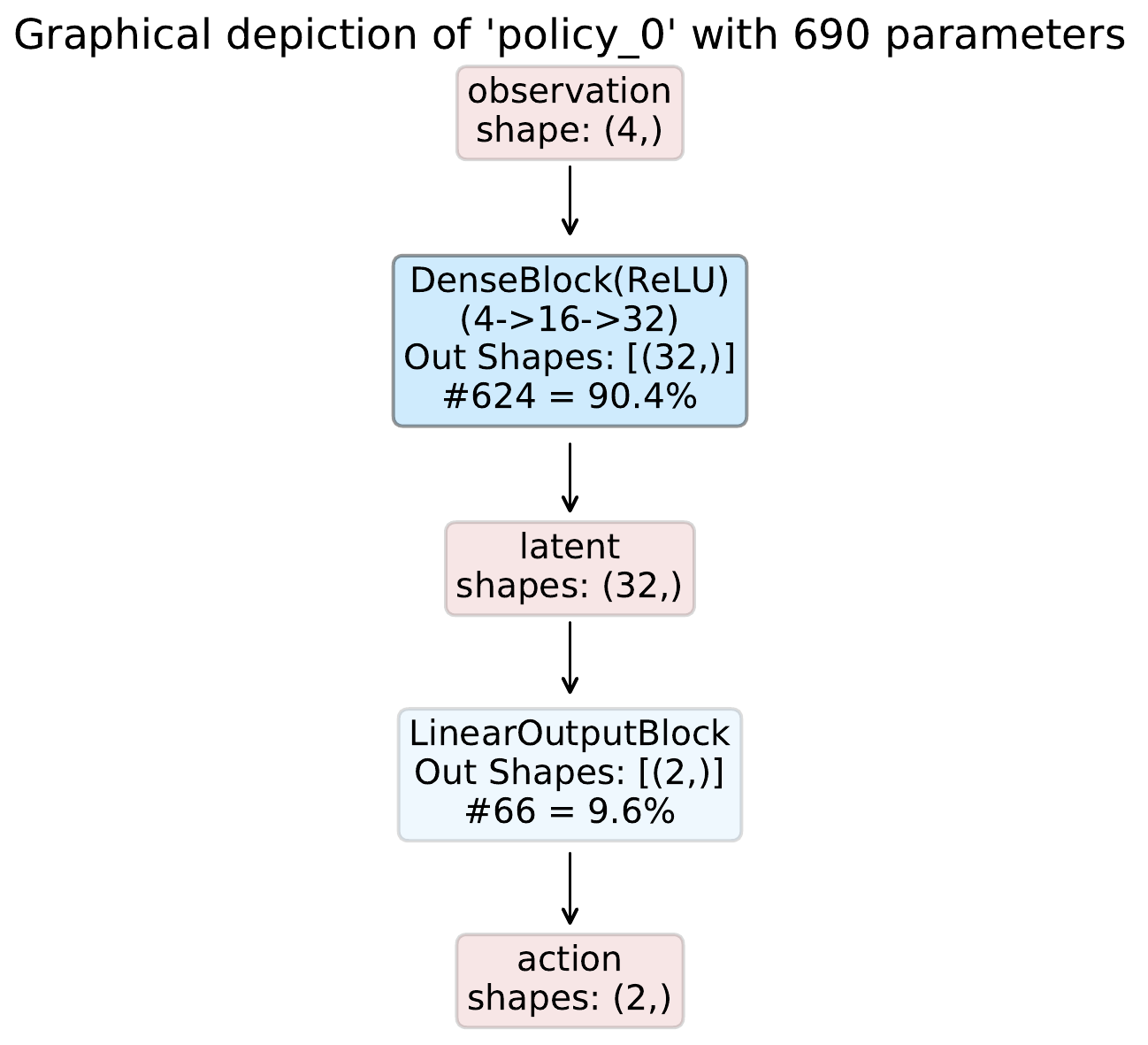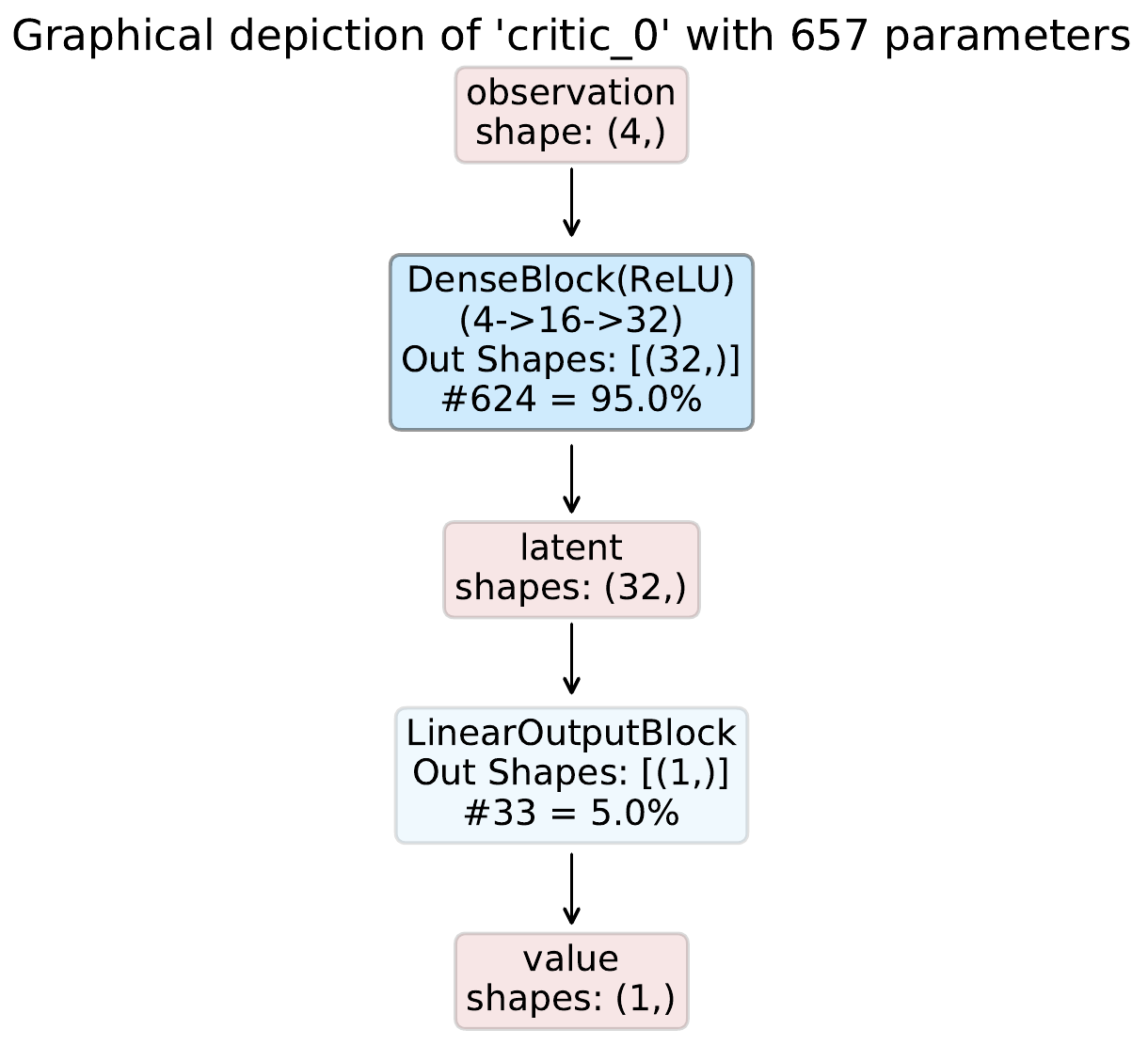Example 2: Complex Networks with Perception Blocks¶

Now we consider the more complex example already used in the template model composer.

The observation space is defined as:

• observation_screen : a 64 x 64 RGB image

• observation_inventory : a 16-dimensional feature vector

The action space is defined as:

• action_move : a categorical action with four options deciding to move [UP, DOWN, LEFT, RIGHT]

• action_use : a 16-dimensional multi-binary action deciding which item to use from inventory

Since we will build a policy and state critic network, where both networks should have the same low level network structure we can create a common base or latent space network:

"""Shows how to use the custom model composer to build a complex custom embedding networks."""
from collections import OrderedDict
from typing import Dict, Union, Sequence, List

import torch.nn as nn

from maze.perception.blocks.feed_forward.dense import DenseBlock
from maze.perception.blocks.general.concat import ConcatenationBlock
from maze.perception.blocks.joint_blocks.lstm_last_step import LSTMLastStepBlock
from maze.perception.blocks.joint_blocks.vgg_conv_dense import VGGConvolutionDenseBlock

class CustomComplexLatentNet:
"""Simple feed forward policy network.

:param obs_shapes: The shapes of all observations as a dict.
:param non_lin: The nonlinear activation to be used.
:param hidden_units: A list of units per hidden layer.
"""

def __init__(self, obs_shapes: Dict[str, Sequence[int]],
non_lin: Union[str, type(nn.Module)], hidden_units: List[int]):
self.obs_shapes = obs_shapes

# Maze relies on dictionaries to represent the inference graph
self.perception_dict = OrderedDict()

# build latent feature embedding block
self.perception_dict['latent_inventory'] = DenseBlock(
in_keys='observation_inventory', out_keys='latent_inventory', in_shapes=obs_shapes['observation_inventory'],
hidden_units=, non_lin=non_lin)

# build latent pixel embedding block
self.perception_dict['latent_screen'] = VGGConvolutionDenseBlock(
in_keys='observation_screen', out_keys='latent_screen', in_shapes=obs_shapes['observation_screen'],
non_lin=non_lin, hidden_channels=[8, 16, 32], hidden_units=)

# Concatenate latent features
self.perception_dict['latent_concat'] = ConcatenationBlock(
in_keys=['latent_inventory', 'latent_screen'], out_keys='latent_concat',
in_shapes=self.perception_dict['latent_inventory'].out_shapes() +
self.perception_dict['latent_screen'].out_shapes(), concat_dim=-1)

self.perception_dict['latent_dense'] = DenseBlock(
in_keys='latent_concat', out_keys='latent_dense', hidden_units=hidden_units, non_lin=non_lin,
in_shapes=self.perception_dict['latent_concat'].out_shapes()
)

self.perception_dict['latent'] = LSTMLastStepBlock(
in_keys='latent_dense', out_keys='latent', in_shapes=self.perception_dict['latent_dense'].out_shapes(),
hidden_size=32, num_layers=1, bidirectional=False, non_lin=non_lin
)

Given this base class we can now create the policy network:

"""Shows how to use the custom model composer to build a complex custom policy networks."""
from typing import Dict, Union, Sequence, List

import numpy as np
import torch
import torch.nn as nn

from docs.source.policy_and_value_networks.code_snippets.custom_complex_latent_net import \
CustomComplexLatentNet
from maze.perception.blocks.inference import InferenceBlock
from maze.perception.blocks.output.linear import LinearOutputBlock
from maze.perception.weight_init import make_module_init_normc

class CustomComplexPolicyNet(nn.Module, CustomComplexLatentNet):
"""Simple feed forward policy network.

:param obs_shapes: The shapes of all observations as a dict.
:param action_logits_shapes: The shapes of all actions as a dict structure.
:param non_lin: The nonlinear activation to be used.
:param hidden_units: A list of units per hidden layer.
"""

def __init__(self, obs_shapes: Dict[str, Sequence[int]], action_logits_shapes: Dict[str, Sequence[int]],
non_lin: Union[str, type(nn.Module)], hidden_units: List[int]):
nn.Module.__init__(self)
CustomComplexLatentNet.__init__(self, obs_shapes, non_lin, hidden_units)

for action_key, action_shape in action_logits_shapes.items():
self.perception_dict[action_key] = LinearOutputBlock(
in_keys='latent', out_keys=action_key, in_shapes=self.perception_dict['latent'].out_shapes(),
output_units=int(np.prod(action_shape)))

# build inference block
in_keys = list(self.obs_shapes.keys())
self.perception_net = InferenceBlock(
in_keys=in_keys, out_keys=list(action_logits_shapes.keys()), perception_blocks=self.perception_dict,
in_shapes=[self.obs_shapes[key] for key in in_keys])

# apply weight init
self.perception_net.apply(make_module_init_normc(1.0))
for action_key in action_logits_shapes.keys():
self.perception_dict[action_key].apply(make_module_init_normc(0.01))

def forward(self, in_tensor_dict: Dict[str, torch.Tensor]) -> Dict[str, torch.Tensor]:
"""Compute forward pass through the network.

:param in_tensor_dict: Input tensor dict.
:return: The computed output of the network.
"""
return self.perception_net(in_tensor_dict)

… and the critic network:

"""Shows how to use the custom model composer to build a complex custom value networks."""
from typing import Dict, Union, Sequence, List

import torch
import torch.nn as nn

from docs.source.policy_and_value_networks.code_snippets.custom_complex_latent_net import \
CustomComplexLatentNet
from maze.perception.blocks.inference import InferenceBlock
from maze.perception.blocks.output.linear import LinearOutputBlock
from maze.perception.weight_init import make_module_init_normc

class CustomComplexCriticNet(nn.Module, CustomComplexLatentNet):
"""Simple feed forward policy network.

:param obs_shapes: The shapes of all observations as a dict.
:param non_lin: The nonlinear activation to be used.
:param hidden_units: A list of units per hidden layer.
"""

def __init__(self, obs_shapes: Dict[str, Sequence[int]],
non_lin: Union[str, type(nn.Module)], hidden_units: List[int]):
nn.Module.__init__(self)
CustomComplexLatentNet.__init__(self, obs_shapes, non_lin, hidden_units)

self.perception_dict['value'] = LinearOutputBlock(
in_keys='latent', out_keys='value', in_shapes=self.perception_dict['latent'].out_shapes(),
output_units=1)

# build inference block
in_keys = list(self.obs_shapes.keys())
self.perception_net = InferenceBlock(
in_keys=in_keys, out_keys='value', in_shapes=[self.obs_shapes[key] for key in in_keys],
perception_blocks=self.perception_dict)

# apply weight init
self.perception_net.apply(make_module_init_normc(1.0))
self.perception_dict['value'].apply(make_module_init_normc(0.01))

def forward(self, in_tensor_dict: Dict[str, torch.Tensor]) -> Dict[str, torch.Tensor]:
"""Compute forward pass through the network.

:param in_tensor_dict: Input tensor dict.
:return: The computed output of the network.
"""
return self.perception_net(in_tensor_dict)

An example config for the model composer could then look like this:

# @package model

# specify the custom model composer by reference
_target_: maze.perception.models.custom_model_composer.CustomModelComposer

# Specify distribution mapping
# (here we use a default distribution mapping)
distribution_mapper_config: []

policy:
_target_: maze.perception.models.policies.ProbabilisticPolicyComposer
networks:
# specify the policy network we would like to use, by reference
- _target_: docs.source.policy_and_value_networks.code_snippets.custom_complex_policy_net.CustomComplexPolicyNet
# specify the parameters of our model
non_lin: torch.nn.ReLU
hidden_units: 
substeps_with_separate_agent_nets: []

critic:
# first specify the critic type (single step in this example)
_target_: maze.perception.models.critics.StateCriticComposer
networks:
# specify the critic we would like to use, by reference
- _target_: docs.source.policy_and_value_networks.code_snippets.custom_complex_critic_net.CustomComplexCriticNet
# specify the parameters of our model
non_lin: torch.nn.ReLU
hidden_units: 

The resulting inference graphs for a recurrent actor-critic model are shown below. Note that the models are identical except for the output layers due to the shared base model.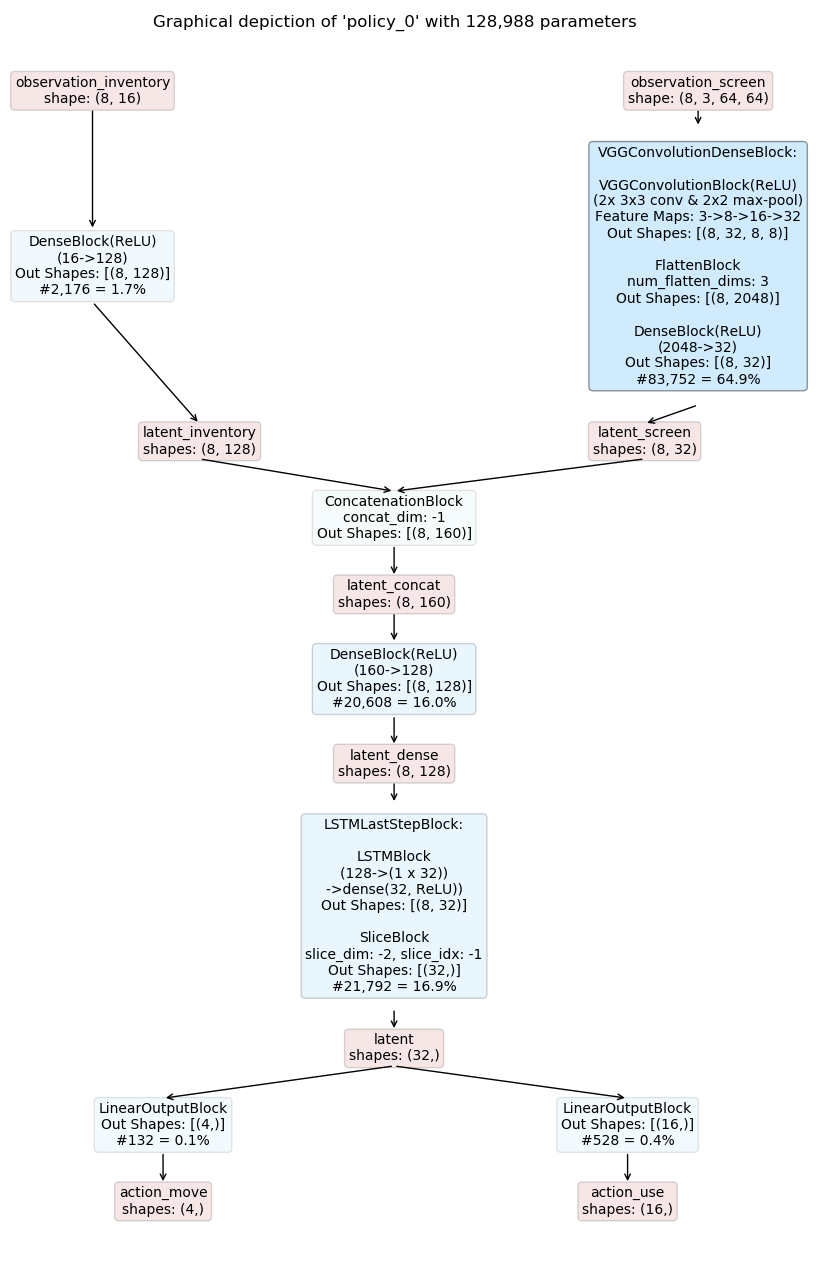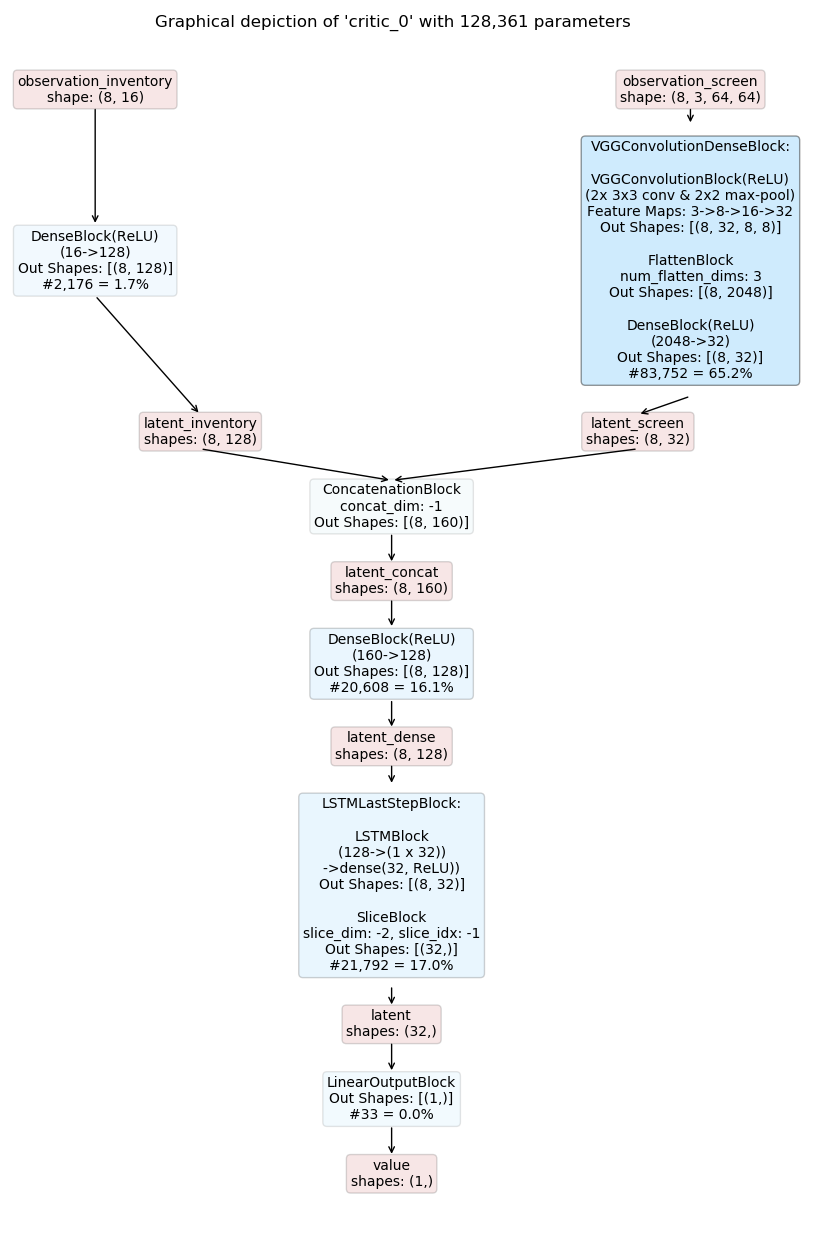Example 3: Custom Networks with (plain PyTorch) Python¶

Here, we take a look at how to create a custom model with plain PyTorch. As already mentioned, we still have to specify the constructor arguments obs_shapes and action_logits_shapes but not necessarily need to use them.

Important: Your models have to use dictionaries with torch.Tensors as values for both inputs and outputs.

For Gym’s CartPole the policy model could be defined like this:

"""Shows how to create a custom cartpole model using no maze perception components."""
from typing import Dict, Sequence

import torch
import torch.nn as nn
import torch.nn.functional as F

class CustomPlainCartpolePolicyNet(nn.Module):
"""Simple feed forward policy network.

:param obs_shapes: The shapes of all observations as a dict.
:param action_logits_shapes: The shapes of all actions as a dict structure.
:param hidden_layer_0: The number of units in layer 0.
:param hidden_layer_1: The number of units in layer 1.
:param use_bias: Specify whether to use a bias in the linear layers.
"""
def __init__(self, obs_shapes: Dict[str, Sequence[int]], action_logits_shapes: Dict[str, Sequence[int]],
hidden_layer_0: int, hidden_layer_1: int, use_bias: bool):
nn.Module.__init__(self)

self.observation_name = list(obs_shapes.keys())
self.action_name = list(action_logits_shapes.keys())

self.l0 = nn.Linear(4, hidden_layer_0, bias=use_bias)
self.l1 = nn.Linear(hidden_layer_0, hidden_layer_1, bias=use_bias)
self.l2 = nn.Linear(hidden_layer_1, 2, bias=use_bias)

def reset_parameters(self) -> None:
"""Reset the parameters of the Model"""

self.l0.reset_parameters()
self.l1.reset_parameters()
self.l1.reset_parameters()

def forward(self, in_tensor_dict: Dict[str, torch.Tensor]) -> Dict[str, torch.Tensor]:
"""Compute forward pass through the network.

:param in_tensor_dict: Input tensor dict.
:return: The computed output of the network.
"""
# Retrieve the observation tensor from the input dict
xx_tensor = in_tensor_dict[self.observation_name]

# Compute the forward pass thorough the network
xx_tensor = F.relu(self.l0(xx_tensor))
xx_tensor = F.relu(self.l1(xx_tensor))
xx_tensor = self.l2(xx_tensor)

# Create the output dictionary with the computed model output
out = dict({self.action_name: xx_tensor})
return out

And the critic model as:

"""Shows how to create a custom cartpole model using no maze perception components."""
from typing import Dict, Sequence

import torch
import torch.nn as nn
import torch.nn.functional as F

class CustomPlainCartpoleCriticNet(nn.Module):
"""Simple feed forward critic network.

:param obs_shapes: The shapes of all observations as a dict.
:param hidden_layer_0: The number of units in layer 0.
:param hidden_layer_1: The number of units in layer 1.
:param use_bias: Specify whether to use a bias in the linear layers.
"""
def __init__(self, obs_shapes: Dict[str, Sequence[int]],
hidden_layer_0: int, hidden_layer_1: int, use_bias: bool):
nn.Module.__init__(self)

self.observation_name = list(obs_shapes.keys())

self.l0 = nn.Linear(4, hidden_layer_0, bias=use_bias)
self.l1 = nn.Linear(hidden_layer_0, hidden_layer_1, bias=use_bias)
self.l2 = nn.Linear(hidden_layer_1, 1, bias=use_bias)

def reset_parameters(self) -> None:
"""Reset the parameters of the Model"""

self.l0.reset_parameters()
self.l1.reset_parameters()
self.l1.reset_parameters()

def forward(self, in_tensor_dict: Dict[str, torch.Tensor]) -> Dict[str, torch.Tensor]:
"""Compute forward pass through the network.

:param in_tensor_dict: Input tensor dict.
:return: The computed output of the network.
"""
# Retrieve the observation tensor from the input dict
xx_tensor = in_tensor_dict[self.observation_name]

# Compute the forward pass thorough the network
xx_tensor = F.relu(self.l0(xx_tensor))
xx_tensor = F.relu(self.l1(xx_tensor))
xx_tensor = self.l2(xx_tensor)

# Create the output dictionary with the computed model output
out = dict({'value': xx_tensor})
return out

An example config for the model composer could then look like this:

# @package model

# specify the custom model composer by reference
_target_: maze.perception.models.custom_model_composer.CustomModelComposer

# Specify distribution mapping
# (here we use a default distribution mapping)
distribution_mapper_config: []

policy:
# first specify the policy type
_target_: maze.perception.models.policies.ProbabilisticPolicyComposer
# specify the policy network(s) we would like to use, by reference
networks:
- _target_: docs.source.policy_and_value_networks.code_snippets.custom_plain_cartpole_policy_net.CustomPlainCartpolePolicyNet
# specify the parameters of our model
hidden_layer_0: 16
hidden_layer_1: 32
use_bias: True
substeps_with_separate_agent_nets: []

critic:
# first specify the critic type (here a state value critic)
_target_: maze.perception.models.critics.StateCriticComposer
# specify the critic network(s) we would like to use, by reference
networks:
- _target_: docs.source.policy_and_value_networks.code_snippets.custom_plain_cartpole_critic_net.CustomPlainCartpoleCriticNet
# specify the parameters of our model
hidden_layer_0: 16
hidden_layer_1: 32
use_bias: True

Note

Since we do not use the inference block in this example, no visual representation of the model can be rendered.

Example 4: Custom Shared embeddings with Perception Blocks¶

For this example we want to showcase the capabilities for using shared embeddings referring to Example 2: for the setup of the observations and actions. Now lets consider the case where we would like to share the observation_screen embedding only.

Here the policy will look very similar to Example 2:, using the same latent net. The only difference here is that we specify an additional out_key when creating the inference block:

"""Shows how to use the custom model composer to build a complex custom policy networks with shared embedding."""
from typing import Dict, Union, Sequence, List

import numpy as np
import torch
import torch.nn as nn

from docs.source.policy_and_value_networks.code_snippets.custom_complex_latent_net import \
CustomComplexLatentNet
from maze.perception.blocks.inference import InferenceBlock
from maze.perception.blocks.output.linear import LinearOutputBlock
from maze.perception.weight_init import make_module_init_normc

class CustomSharedComplexPolicyNet(nn.Module, CustomComplexLatentNet):
"""Simple feed forward policy network.

:param obs_shapes: The shapes of all observations as a dict.
:param action_logits_shapes: The shapes of all actions as a dict structure.
:param non_lin: The nonlinear activation to be used.
:param hidden_units: A list of units per hidden layer.
"""

def __init__(self, obs_shapes: Dict[str, Sequence[int]], action_logits_shapes: Dict[str, Sequence[int]],
non_lin: Union[str, type(nn.Module)], hidden_units: List[int]):
nn.Module.__init__(self)
CustomComplexLatentNet.__init__(self, obs_shapes, non_lin, hidden_units)

for action_key, action_shape in action_logits_shapes.items():
self.perception_dict[action_key] = LinearOutputBlock(
in_keys='latent', out_keys=action_key, in_shapes=self.perception_dict['latent'].out_shapes(),
output_units=int(np.prod(action_shape)))

# build inference block
in_keys = list(self.obs_shapes.keys())
# Specifically add 'latent_screen' as an out_key to the network, so it will get returned when calling the
#   forward method and can be reused by the critic network.
out_keys = list(action_logits_shapes.keys()) + ['latent_screen']
self.perception_net = InferenceBlock(
in_keys=in_keys, out_keys=out_keys,
perception_blocks=self.perception_dict,
in_shapes=[self.obs_shapes[key] for key in in_keys])

# apply weight init
self.perception_net.apply(make_module_init_normc(1.0))
for action_key in action_logits_shapes.keys():
self.perception_dict[action_key].apply(make_module_init_normc(0.01))

def forward(self, in_tensor_dict: Dict[str, torch.Tensor]) -> Dict[str, torch.Tensor]:
"""Compute forward pass through the network.

:param in_tensor_dict: Input tensor dict.
:return: The computed output of the network.
"""
return self.perception_net(in_tensor_dict)

Now for the critic, we already get the specified additional out_key as part of the obs_shapes dict and can therefore be used as such:

"""Shows how to use the custom model composer to build a complex custom value networks with shared embedding."""
from collections import OrderedDict
from typing import Dict, Union, Sequence, List

import torch
import torch.nn as nn

from maze.perception.blocks.feed_forward.dense import DenseBlock
from maze.perception.blocks.general.concat import ConcatenationBlock
from maze.perception.blocks.inference import InferenceBlock
from maze.perception.blocks.joint_blocks.lstm_last_step import LSTMLastStepBlock
from maze.perception.blocks.output.linear import LinearOutputBlock
from maze.perception.weight_init import make_module_init_normc

class CustomSharedComplexCriticNet(nn.Module):
"""Simple feed forward policy network.

:param obs_shapes: The shapes of all observations as a dict.
:param non_lin: The nonlinear activation to be used.
:param hidden_units: A list of units per hidden layer.
"""

def __init__(self, obs_shapes: Dict[str, Sequence[int]],
non_lin: Union[str, type(nn.Module)], hidden_units: List[int]):
nn.Module.__init__(self)

# Maze relies on dictionaries to represent the inference graph
self.perception_dict = OrderedDict()

# build latent feature embedding block
self.perception_dict['latent_inventory'] = DenseBlock(
in_keys='observation_inventory', out_keys='latent_inventory', in_shapes=obs_shapes['observation_inventory'],
hidden_units=, non_lin=non_lin)

# Concatenate latent features
self.perception_dict['latent_concat'] = ConcatenationBlock(
in_keys=['latent_inventory', 'latent_screen'], out_keys='latent_concat',
in_shapes=self.perception_dict['latent_inventory'].out_shapes() +
[obs_shapes['latent_screen']], concat_dim=-1)

self.perception_dict['latent_dense'] = DenseBlock(
in_keys='latent_concat', out_keys='latent_dense', hidden_units=hidden_units, non_lin=non_lin,
in_shapes=self.perception_dict['latent_concat'].out_shapes()
)

self.perception_dict['latent'] = LSTMLastStepBlock(
in_keys='latent_dense', out_keys='latent', in_shapes=self.perception_dict['latent_dense'].out_shapes(),
hidden_size=32, num_layers=1, bidirectional=False, non_lin=non_lin
)

self.perception_dict['value'] = LinearOutputBlock(
in_keys='latent', out_keys='value', in_shapes=self.perception_dict['latent'].out_shapes(),
output_units=1)

# build inference block
in_keys = list(obs_shapes.keys())
self.perception_net = InferenceBlock(
in_keys=in_keys, out_keys='value', in_shapes=[obs_shapes[key] for key in in_keys],
perception_blocks=self.perception_dict)

# apply weight init
self.perception_net.apply(make_module_init_normc(1.0))
self.perception_dict['value'].apply(make_module_init_normc(0.01))

def forward(self, in_tensor_dict: Dict[str, torch.Tensor]) -> Dict[str, torch.Tensor]:
"""Compute forward pass through the network.

:param in_tensor_dict: Input tensor dict.
:return: The computed output of the network.
"""
return self.perception_net(in_tensor_dict)

The yaml file would look the same as for Example 2:, we only specify different networks.

# @package model

# specify the custom model composer by reference
_target_: maze.perception.models.custom_model_composer.CustomModelComposer

# Specify distribution mapping
# (here we use a default distribution mapping)
distribution_mapper_config: []

policy:
_target_: maze.perception.models.policies.ProbabilisticPolicyComposer
networks:
# specify the policy network we would like to use, by reference
- _target_: docs.source.policy_and_value_networks.code_snippets.custom_shared_complex_policy_net.CustomSharedComplexPolicyNet
# specify the parameters of our model
non_lin: torch.nn.ReLU
hidden_units: 
substeps_with_separate_agent_nets: []

critic:
# first specify the critic type (single step in this example)
_target_: maze.perception.models.critics.StateCriticComposer
networks:
# specify the critic we would like to use, by reference
- _target_: docs.source.policy_and_value_networks.code_snippets.custom_shared_complex_critic_net.CustomSharedComplexCriticNet
# specify the parameters of our model
non_lin: torch.nn.ReLU
hidden_units: 

The resulting inference graphs for a recurrent shared actor-critic model are shown below.